International Journal of Transportation Engineering and Technology
Volume 2, Issue 1, March 2016, Pages: 8-14

Determination of Added Mass and Inertia Moment of Marine Ships Moving in 6 Degrees of Freedom

Do Thanh Sen1, Tran Canh Vinh2

1Maritime Education and Human Resource Center (UT-STC), Ho Chi Minh City, Vietnam

2Faculty of Navigation, Ho Chi Minh City University of Transport, Ho Chi Minh City, Vietnam

Email address: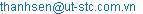(D. T. Sen)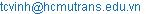(T. C. Vinh)

To cite this article:

Do Thanh Sen, Tran Canh Vinh. Determination of Added Mass and Inertia Moment of Marine Ships Moving in 6 Degrees of Freedom. International Journal of Transportation Engineering and Technology. Vol. 2, No. 1, 2016, pp. 8-14. doi: 10.11648/j.ijtet.20160201.12

Received: March 16, 2016; Accepted: March 30, 2016; Published: April 25, 2016

Abstract: When a ship moves in water with acceleration or deceleration, quantities of fluid moving around the hull creating additional hydrodynamic forces acting on the hull. It is imagined as the added mass which increases the total system mass and inertia moment. In order to establish the mathematical model for ship motion, the added components need to be determined. For a particular ship, these hydrodynamic components can be obtained by experiment. However, for ship simulation especially at the initial design stage it is necessary to calculate and estimate by theoretical method. This study aims to find out a general method to calculate all components of added mass and inertia moment in 6 degrees of freedom for simulating ship movement.

Keywords: Added Mass, Hydrodynamic Coefficient, Mathematical Modeling, Ship Simulation

1. Introduction

According to potential flow theory, no force exists when a rigid body moves at a steady speed through ideal fluid. However, once it accelerates, an hydrodynamic force experiences proportionally to the acceleration. The hydrodynamic force (FH) can be described :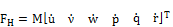(1.1)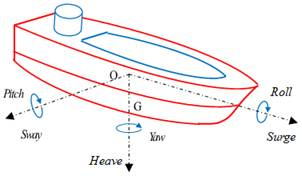Figure 1. Motions in six degrees of freedom (6DOF).

Where,is rigid-body velocity matrix in 6 degrees of freedom (6DOF) (see fig. 1 and table 1):

Table 1. Table of degrees of freedom DOF.

 DOF description velocities 1 surge - motion in x direction u Linear velocities 2 sway - motion in y direction v 3 heave - motion in z direction w 4 roll – rotation about the x axis p Angular velocities 5 pitch - rotation about the y axis q 6 yaw - rotation about the z axis r

M is system inertia matrix of the rigid body and added mass. When a ship accelerates or decelerates on water, quantities of surrounding water moving together with the hull increase the system inertia matrix:

M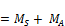(1.2)

Where MS is mass and inertia moment matrix of the ship which is derived: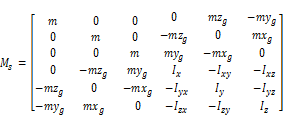(1.3)

MA is added mass and added inertia moment matrix. Assuming that mij is a component in the ith direction caused by acceleration in direction j. It is defined as hydrodynamic force due to unit acceleration and consists of 36 components: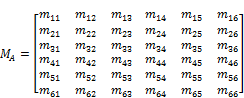(1.4)

Added mass was first introduced by Dubua in 1776  then was expressed mathematically and exactly by Green in 1883 and Stokes in 1843  by expression of added mass of a sphere. Later many researchers generalized the notion of added mass to an arbitrary body moving in different regimes .

In the past, there were many studies determining the added mass including experiment and theoretical prediction.

For a particular ship, it can be obtained by experimental method. However, the experimental method is limited and cannot solve all added mass components. For simulating the ship motion especially in initial design stage, M has to be calculated by estimation method.

The principal for calculating of added mass is based on work of Ursell in 1949  and Frank in 1967  for arbitrary symmetric cross section. Then Keil introduced method for any arbitrary water depth based on a variation of the method of Ursell with Lewis conformal mapping in 1974 . In 1967, Frank described the pulsating source method for deep water .

Nils Salvesen, E. O. Tuck and Odd Faltisen introduced new method to predict heave, pitch, sway, roll and yaw motions as well as wave-induced vertical and horizontal shear forces, bending moments, and torsional moments for a ship advancing at constant speed with arbitrary heading in regular waves in 1970 .

Previous studies showed that mij could not be determined by a unique method. A total way to determine all components of added mass and added moment of inertia is necessary. It is suggested to combine some methods to determine all the remaining components. This study aims to generalize and propose an optimal combination of different methods to calculate total components of the added mass matrix with computer.

2. Methods to Determine Added Mass

2.1. Elimination of Added Mass Components Due to Symmetry of Ship Hull and Added Mass Matrix

With marine ships, the body is symmetric on port-starboard (xy plane), it can be concluded that vertical motions due to heave and pitch induce no transversal force:

m32 = m34 = m36 = m52 = m54 = m56 = 0.

Due to the symmetry of the added mass matrix, it can concluded that mij = mji,:

m23 = m43 = m63 = m25 = m45 = m65 = 0.

The same consideration is applied for the longitudinal motions caused by acceleration in direction j = 2, 4, 6:

m12 = m14 = m16 = 0, and

m21 = m41 = m61 = 0

Thus, for a ship moving in 6DOF, 36 components of added mass are reduced to 18: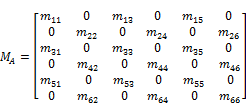(2.1)

The problem is to determine all remaining components of the matrix MA by predictive method.

2.2. Method of Equivalent Ellipsoid

Basing on theory of kinetic energy of fluid mij is determined from the formula: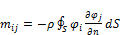(2.2)

Where S is the wetted ship area, r is water density,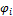is potentials of the flow when the ship is moving in ithdirection with unit speed. Potentialssatisfy the Laplace equation .

To calculate mij, the ship can be relatively assumed as a 3D body such as sphere, spheroid, ellipsoid, rectangular, cylinder etc. (fig. 2)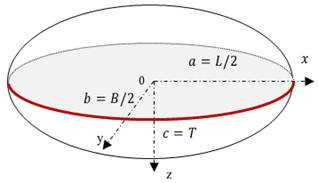Figure 2. Ship assumed as an Ellipsoid

With a marine ship, the most representative of the hull is elongated ellipsoid with c/b = 1 and r = a/b. Where a, b are semi axis of the ellipsoid. Basing on theory of hydrostatics, m11, m22, m33, m44, m55, m66 can be described , :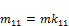(2.3)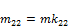(2.4)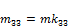(2.5)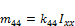(2.6)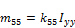(2.7)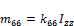(2.8)

For simplifying the calculation, each component can be represented by correspondent kij called hydrodynamic coefficient.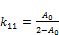(2.9)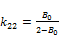(2.10)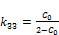(2.11)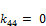(2.12)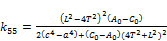(2.13)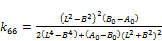(2.14)

where: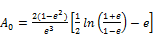(2.15)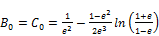(2.16)

with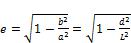(2.17)

d and L are maximum diameter and length overall. Inertia moment of the displaced water is approximately the moment of inertia of the equivalent ellipsoid: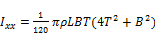(2.18)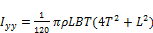(2.19)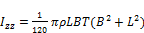(2.20)

The limitation of this method is that the calculating result is only an approximation. The more equivalent to the elongated ellipsoid it is, the more accurate the result is obtained. Moreover, this method cannot determine component m24; m26, m35; m44, m15 and m51.

2.3. Strip Theory Method

The ''Ordinary Strip Theory Method" was introduced by Korvin-Kroukovsky and Jacobs in 1957. Then it was developed by Tasai in 1969 with a "Modified Strip Theory Method" .

Basing on this method a ship can be made up of a finite number of transversal 2D slices (fig. 3). Each slice has a form closely resembling the segment of the representative ship and its added mass can be easily calculated. The added mass of the whole ship is obtained by integration of the 2D value over the length of the hull. Components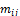are determined: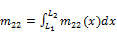(2.21)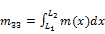(2.22)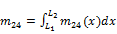(2.23)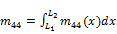(2.24)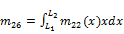(2.25)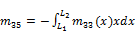(2.26)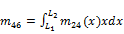(2.27)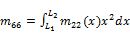(2.28)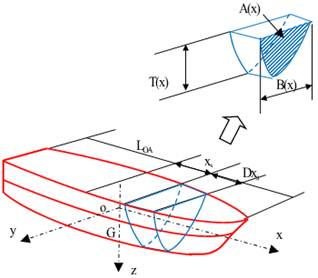Figure 3. The hull is divided into slices.

Where mij(x) is added mass of 2D cross section at location xs.

Lewis transformation mapping:

In practice the form of each slice is various and complex. For numbering and calculating in computer Lewis transformation is the most proper solution.

With this method a cross section of hull is mapped conformably to the unit semicircle (ζ-plane) which is derived in details in , , ,  (fig. 4):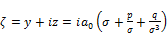(2.29)

and the unit semicircle is derived: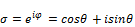(2.30)

Where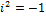;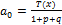. By substituting into the formula (2.15), descriptive parameters of a cross section can be obtained: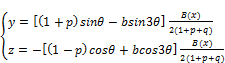(2.31)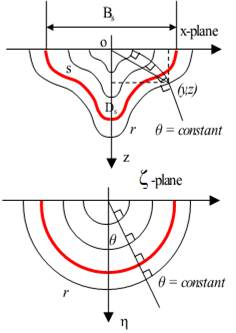Figure 4. Mapping relation between x- and ζ –plane.

Where B(x), T(x) are the breadth and draft of the cross section s. Parameter p, q are described by means of the ratio H(x) and β(x).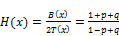(2.32)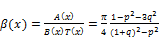(2.33)

Parameter θ is physical meaningless. It corresponds to the polar angle of given point prior to conformal transformation from a semicircle: π/2 ≥ θ ≥ -π/2.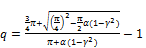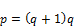(2.34)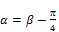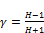(2.35)

The component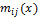of each section is determined by formulas: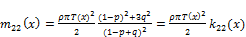(2.36)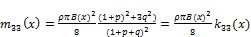(2.37)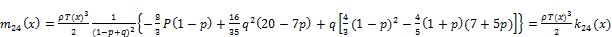(2.38)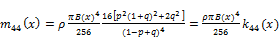(2.39)

Then, total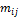is calculated: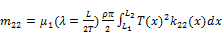(2.40)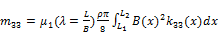(2.41)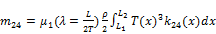(2.42)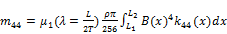(2.43)(2.44)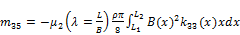(2.45)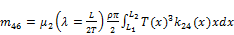(2.46)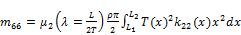(2.47)

Where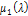,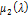are corrections related to fluid motion along x-axis due to elongationof the body .is correction for the added mass which can be used the Pabst correction as the most well-known experimental correction: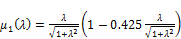(2.48)is correction for the added moment of inertia using the theoretical formula: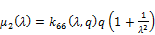(2.49)

It also necessary to note that specific forms of ships consisting of re-entrant forms and asymmetric forms are not acceptable for applying Lewis forms , .

To have a section accepted for Lewis forms, the area coefficient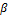is bounded by a lower limit to omit re-entrant Lewis forms and by an upper limit to omit asymmetric Lewis forms:

For H(x) ≥ 1.0: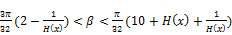(2.50)

For H(x) ≤ 1.0: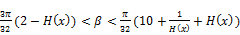(2.51)

2.4. Determining Remaining Added Mass

It is understandable that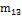are relatively small in comparison with total added mass and can be ignored. Thus,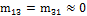.

The Ellipsoid and Strip theory methods do not determine component m15. It is approximately considered that the component m15 and m24 are caused by the hydrodynamic force due to m11 and m22 with the force center at the center of buoyancy of the hull ZB . Therefore: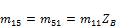(2.52)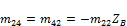(2.53)

Thus, the formula to calculate m15 and m51 is obtained: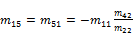(2.54)

When m24 and m42 can be obtained by the Strip theory method.

3. Calculation and Assessment

For comparison and assessment of results, the ship model experimented by Motora  (see fig. 5) is used , :

L: 170.0m

B: 22.8m

T: 9.3m

Displacement: 20.876 MT

The ship is divided longitudinally into 20 slices with ratio B(x)/2T(x) and A(x)/B(x) as in table 2:

Table 2. Sectional presentation of H(x) and β(x).

 Slice Sta dx X H=B/2T β=A/B 21 10.000 4.146 95.366 0.000 0.000 20 9.750 4.146 91.220 0.160 0.540 19 9.500 4.146 87.073 0.275 0.562 18 9.250 4.146 82.927 0.366 0.548 17 9.000 8.293 74.634 0.448 0.556 16 8.500 8.293 66.341 0.650 0.669 15 8.000 16.585 49.756 0.860 0.679 14 7.000 16.585 33.171 1.136 0.782 13 6.000 16.585 16.585 1.226 0.870 12 5.000 16.585 0.000 1.226 0.921 11 4.000 16.585 -16.585 1.226 0.885 10 3.000 16.585 -33.171 1.211 0.774 9 2.000 8.293 -41.463 0.980 0.674 8 1.500 8.293 -49.756 0.799 0.529 7 1.000 4.146 -53.902 0.605 0.410 6 0.750 4.146 -58.049 0.486 0.440 5 0.500 4.146 -62.195 0.404 0.470 4 0.250 4.146 -66.341 0.299 0.500 3 0.000 2.073 -68.415 0.910 0.572 2 -0.125 2.073 -70.488 1.028 0.500 1 -0.250 0.000 -70.488 0.000 0.000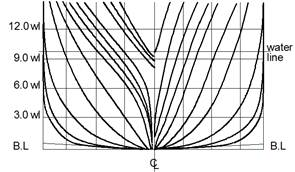Figure 5. Actual body plan of the sample ship.

Numbering values of mapping are calculated and displayed in curves on computer in figure 6, 7 and 8. The results indicate that the transformation is relatively proper in comparison with the actual forms.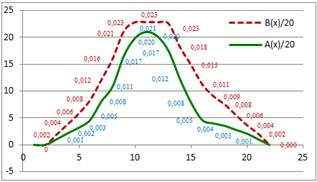Figure 6. B(x) and A(x).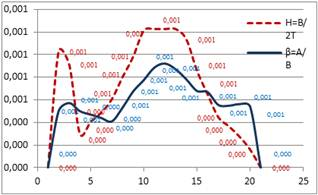Figure 7. H(x) and β(x).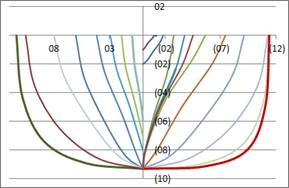Figure 8. Body plan presented using Lewis forms.

The calculating results of above suggested methods and experimental data are summed up and presented in table 3. The column "proposed" is the value suggested for application.

Table 3. Comparing results of mij.

 mij Ellipsoid Lewis experiment Proposed m11/m 0.033 0.032 0.033 m22/m 0.939 0.986 1.040 0.986 m33/m 0.939 1.004 1.004 m44/mB2 0.010 0.010 m55/mL2 0.039 0.039 m66/mL2 0.039 0.045 0.056 0.045 m24/m 0.628 0.628 m26/mL 0.023 0.023 m35/mB -0.042 -0.042 m46/mL 0.107 0.107 m15/m -0.021 -0.021

Basing on the above results, it is concluded that Strip theory method with Lewis forms can determine most component mij with high accuracy due to equivalent transformation. However, this method cannot determine component m11, m55 which can be solved by considering the ship as an elongated ellipsoid.

As for the component m15 = m51, this value is not so high, the estimation in the formula (2.54) is satisfied and acceptable.

4. Suggestion

All components of added mass and added moment of inertia can be calculated by combination of different methods. This study suggests a method to determine all components mij of the added mass matrix as in table 4 below:

Table 4. Suggestion for determining mij.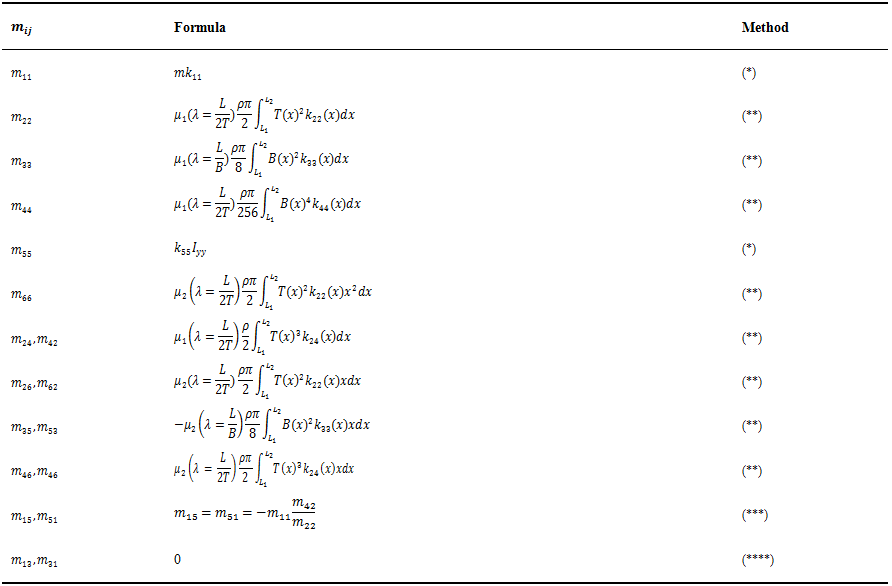(*) determined by assuming the ship as an equivalent elongated ellipsoid.

(**) determined by "Strip theory" with Lewis transformation.

(***) determined by formula (2.54).

(****) can be ignored.

5. Conclusion

In order to determine added mass and added moment of inertia, various methods are necessary to use for particular groups of component mij. The suggested combination method can determine all mij necessary to establish the mathematical model for motion of marine ships in 6DOFs used for computerizing simulation especially at the initial design stage.

However, for a hull with port-starboard asymmetry or re-entrant forms the Lewis shape of ship frame is inapplicable, further assessment for proper calculation should be taken into consideration for obtaining satisfied results.

References

1. THOR I. FOSSEN, Handbook of Marine Craft Hydrodynamics and Motion Control, Norwegian University of Science and Technology Trondheim, Norway, John Wiley & Sons, 2011.
2. EDWARD M. LEWANDOWSKI, The Dynamics Of Marine Craft, Manoeuvring and Seakeeping, World Scientific, Vol 22,2004,pp. 35-54.
3. HABIL. NIKOLAI KORNEV, Lectures on ship manoeuvrability, Rostock University Universität Rostock, Germany, 2013.
4. ALEXANDR I. KOROTKIN, Added Masses of Ship Structures, Krylov Shipbuilding Research Institute - Springer, St. Petersburg, Russia,2009,pp. 51-55, pp. 86-88, pp. 93-96.
5. J.P.HOOFT, "The Prediction of the Ship’s Manoeuvrability in the Design Stage", SNAME transaction, Vol. 102,1994,pp. 419-445.
6. J.M.J. JOURNÉE & L.J.M. ADEGEEST, Theoretical Manual of Strip Theory Program "SEAWAY for Windows", Delft University of Technology, the Netherlands,2003,pp. 53-56.
7. TRAN CONG NGHI, Ship theory – Hull resistance and Thrusters (Volume II), Ho Chi Minh city University of Transport,2009,pp. 208-222.
8. NILS SALVESEN, E. O. TUCK và ODD FALTISEN, Ship Motions and Sealoads, The Society of Naval Architects and Marine Engineers, No. 6, 1970.
9. BIRKHOFF, G., Hydrodynamics, Princeton University Press, Princeton, 1960.
10. LAMB, G., Hydrodynamics, Cambridge University Press, Cambridge, 1932.
11. F. URSELL, On the Heaving Motion of a Circular Cylinder on the Surface of Fluid, Quarterly Journal of Mechanics and Applied Mathematics, Vol II, 1949.
12. W. Frank, Oscillation of Cylinders in or below the Free Surface of Deep Fluids, Technical Report 2375, 1967, Naval Ship Research and Development Centre, Washington DC, USA, 1967.
13. H. KEIL, Die hydrodynamischen Kräfte bei der periodischen Bewegung zweidimensionaler Körper an der Oberfläche flacher Gewässer, Ber. Nr. 305. Institut für Schiffbau. Univ. Hamburg, Deutschland.
14. Seizo Motora,On the Measurement of Added Mass and Added Moment of Inertia for ship Motions,Journal of Zosen Kiokai, No. 107, 1960.

Biography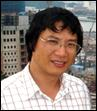Do Thanh Sen (1972, Ha Noi), Master Mariner; Attorney at law. He currently is a navigation lecturer and has been conducting many projects in Bridge simulator training, Maritime consultancy and applied research at the Maritime Education and Human Resource center as a member of STC-Group, the Netherlands. His research interests are Ship mathematical modelling, Marine simulators, Maritime education, IT and Maritime law. He authored 2 books, 7 publications in scientific papers and 3 maritime computer applications.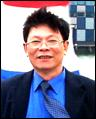Tran Canh Vinh (1951, Nghe An), PhD Thesis on speciality of Control of technical systems (1999, Russia), Associate Prof. in 2005. He is currently Senior Lecturer in Ho Chi Minh City University of Transport (Vietnam) in Automation control, Electronic navigation aids. His research interest includes Control of ships and Electronic navigation. He has authored 3 books and 30 publications in scientific papers and many presentations on national and international conferences.
 Contents 1. 2. 2.1. 2.2. 2.3. 2.4. 3. 4. 5.
Article ToolsAbstractPDF(1654K)
Follow on us
PUBLICATION SERVICE
JOIN US
RESOURCES
SPECIAL SERVICES
ADDRESS
Science Publishing Group
548 FASHION AVENUE
NEW YORK, NY 10018
U.S.A.
Tel: (001)347-688-8931